arrow_back

# TensorFlow: Qwik Start

40 minutes 1 Credit

## GSP637## Overview

In this lab you will learn the basic ‘Hello World' of machine learning where, instead of programming explicit rules in a language such as Java or C++, you build a system that is trained on data to infer the rules that determine a relationship between numbers.

### What you'll do

• Set up the development environment in the IDE

• Design a Machine Learning Model

• Train the Neural Network

• Test the model

## Setup and requirements

### Before you click the Start Lab button

Read these instructions. Labs are timed and you cannot pause them. The timer, which starts when you click Start Lab, shows how long Google Cloud resources will be made available to you.

This hands-on lab lets you do the lab activities yourself in a real cloud environment, not in a simulation or demo environment. It does so by giving you new, temporary credentials that you use to sign in and access Google Cloud for the duration of the lab.

To complete this lab, you need:

Note: Use an Incognito or private browser window to run this lab. This prevents any conflicts between your personal account and the Student account, which may cause extra charges incurred to your personal account.
• Time to complete the lab---remember, once you start, you cannot pause a lab.
Note: If you already have your own personal Google Cloud account or project, do not use it for this lab to avoid extra charges to your account.

## Introduction

Consider the following problem: you're building a system that performs activity recognition for fitness tracking. You might have access to the speed at which a person is moving and attempt to infer their activity based on this speed using a conditional:

if(speed<4){ status=WALKING; }
• You could extend this to running with another condition:
if(speed<4){ status=WALKING; } else { status=RUNNING; }
• Similarly, you could detect cycling with another condition:
if(speed<4){ status=WALKING; } else if(speed<12){ status=RUNNING; } else { status=BIKING; }
• Now consider what happens when you want to include an activity like golf? Now, it becomes less obvious how to create a rule to determine the activity.
// Now what?

It's extremely difficult to write a program (expressed in code) that helps you detect the golfing activity.

So what do you do? You can use machine learning to solve the problem!

## What is machine learning?

In the previous section you encountered a problem when you tried to determine a user's fitness activity. You hit limitations in what you could achieve by writing more code since your conditions have to be more complex to detect an activity like golf.

Consider building applications in the traditional manner as represented in the following diagram: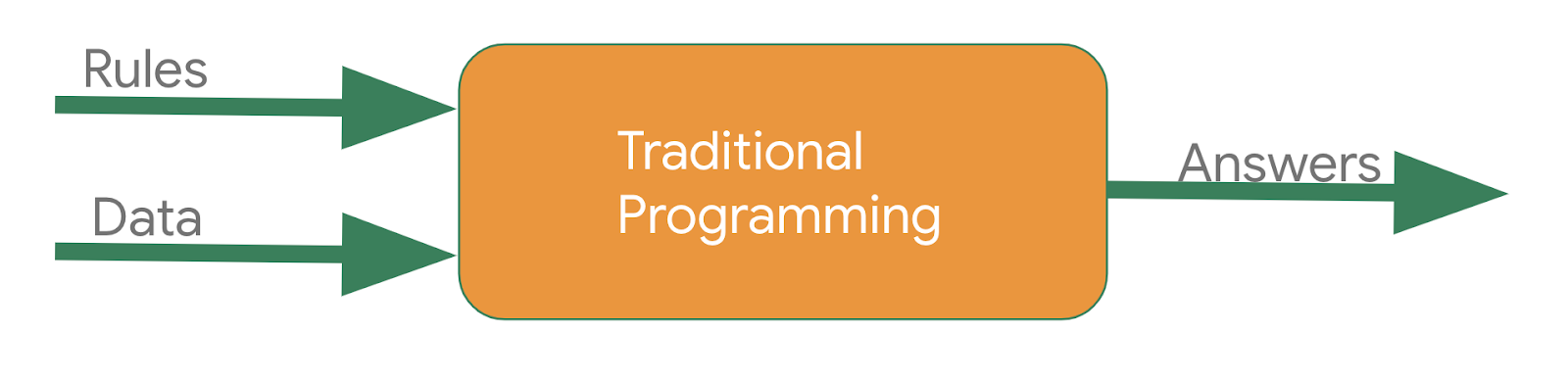You express rules in a programming language. These act on data and your program provides answers. In the case of activity detection, the rules (the code you wrote to define types of activities) acted upon the data (the person's movement speed) in order to find an answer -- the return value from the function for determining the activity status of the user (whether they were walking, running, biking, etc.).

The process for detecting this activity via machine learning is very similar -- only the axes are different: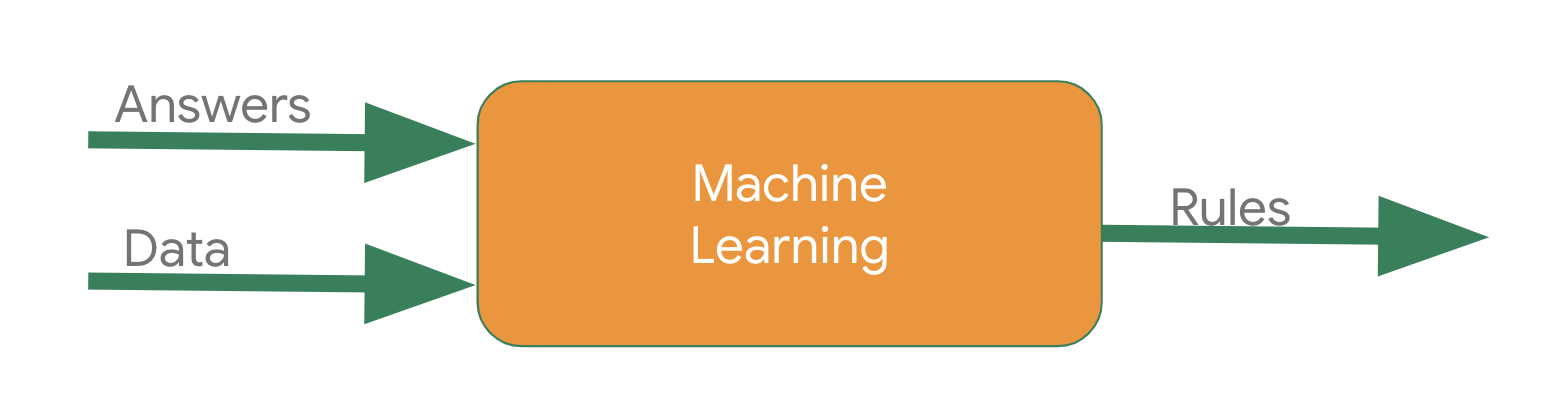Instead of trying to define the rules and expressing them in a programming language, you provide the answers (typically called labels) along with the data. The machine then infers the rules that determine the relationship between the answers and the data. For example, in a machine learning context, your activity detection scenario might look like this: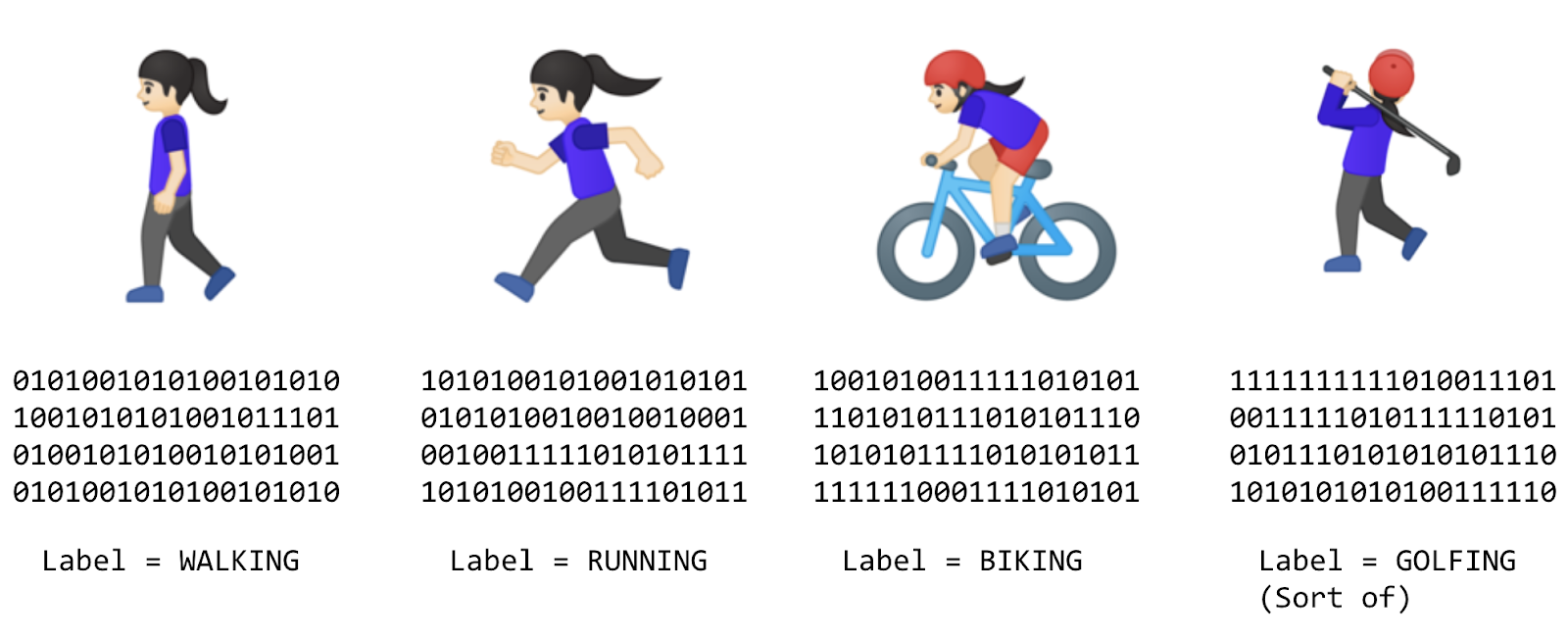You gather lots of data, and label it to effectively say "This is what walking looks like", "This is what running looks like" etc. Then, from the data, the computer can infer the rules that determine what the distinct patterns that denote a particular activity are.

Beyond being an alternative method to programming this scenario, this also gives you the ability to open up new scenarios, such as golfing, which may not have been possible under the traditional rule-based programming approach.

In traditional programming your code compiles into a binary that is typically called a program. In machine learning, the item that you create from the data and labels is called a model.

So if you go back to this diagram:Consider the result of the above to be a model, which is used like this at runtime: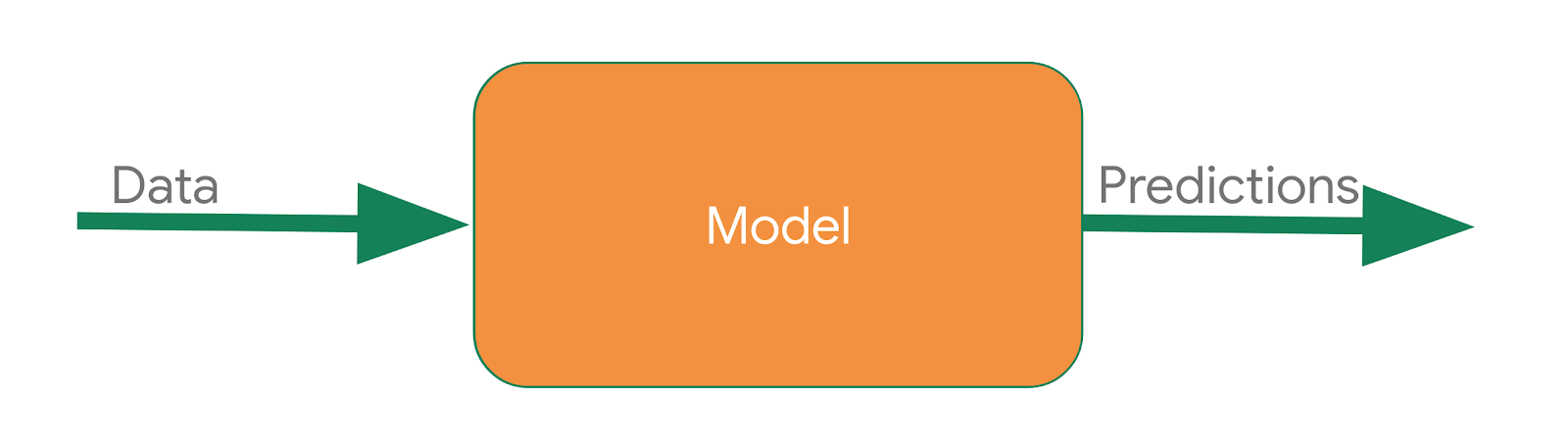You pass the model some data, and the model uses the rules it inferred from the training to come up with a prediction -- i.e. "That data looks like walking", "That data looks like biking" etc.

In this lab you will build a very simple ‘Hello World' model made up of the building blocks that can be used in any machine learning scenario!

## Task 1. Access Cloud Code

In this lab, you will be using Cloud Code for creating and running python scripts.

Cloud Code is a set of IDE plugins for popular IDEs that make it easier to create, deploy and integrate applications with Google Cloud.

A Cloud Code development environment has already been set up to make things easier for you.

1. Copy the `IDE` URL from the lab panel.

2. Paste it into a new browser window.

Note: The lab environment is based on a development environment. In this environment, you can run and test the python/tensorflow script from the IDE.

### Check for required packages in the IDE environment

1. Open a terminal in the IDE and navigate to `Application Menu` > `Terminal` > `New Terminal`.1. Check if your Python environment is already configured. Copy and paste the following command in the terminal:

python --version Note: Incase you get a browser popup while pasting the command in the IDE terminal, click `Allow` on the popup to enable copy/paste in the IDE.

Example output:

xx:xx:xx ide-dev@cloudlearningservices ~ → python --version Python 3.8.1
1. Check if TensorFlow is installed. Run the following command in the terminal:
python -c "import tensorflow;print(tensorflow.__version__)"

Example output:

xx:xx:xx ide-dev@cloudlearningservices ~ → python -c "import tensorflow;print(tensorflow.__version__)" 2.8.0

The output shows that Python 3.8.1 and TensorFlow 2.8.0 are already installed in your IDE environment.

• Consider the following sets of numbers. Can you see the relationship between them?
 X: -1 0 1 2 3 4 Y: -2 1 4 7 10 13

As you read left to right, notice that the X value is increasing by 1 and the corresponding Y value is increasing by 3. So, the relationship should be Y=3X plus or minus some value.

Then, take look at the 0 on X and see that the corresponding Y value is 1.

From both of these observations, you can determine that the relationship is Y=3X+1.

This is almost exactly how you would use code to train a model, known as a neural network, to spot the patterns in the data!

You use data to train the neural network! By feeding it with a set of Xs and a set of Ys, it should be able to figure out the relationship between them.

### Create a new file.

• Navigate to `Application Menu` > `File` > `New File`

### Import necessary packages

1. Import and configure `logging` and `google-cloud-logging` for cloud logging. Add the following code to the file:

1. Import `tensorflow` for training and evaluating the model. Call it `tf` for ease of use. Add the following code to the file:

# Import TensorFlow import tensorflow as tf
1. Import `numpy`, to represent the data as lists. Call it `np` for ease of use. Add the following code to the file:

# Import numpy import numpy as np

### Prepare the data

Next up, you will prepare the data your model will be trained on. In this lab, you're using the 6 Xs and 6 Ys used earlier:

 X: -1 0 1 2 3 4 Y: -2 1 4 7 10 13

As you can see, the relationship between the Xs and Ys is Y=3x+1, so where X = 1, Y = 4 and so on.

A python library called `numpy` provides lots of array type data structures that are a defacto standard way of feeding in data. To use these, specify the values as an array in `numpy` using `np.array([])`

• Add the following code to the file:

xs = np.array([-1.0, 0.0, 1.0, 2.0, 3.0, 4.0], dtype=float) ys = np.array([-2.0, 1.0, 4.0, 7.0, 10.0, 13.0], dtype=float)

### Design the model

In this section, you will design your model using TensorFlow.

You will use a machine learning algorithm called neural network to train your model. You will create the simplest possible neural network. It has 1 layer, and that layer has 1 neuron. The neural network's input is only one value at a time. Hence, the input shape must be .

• Add the following code to the file:

model = tf.keras.Sequential([tf.keras.layers.Dense(units=1, input_shape=)])

### Compile the model

Next, you will write the code to compile your neural network. When you do, you must specify 2 functions, a `loss` and an `optimizer`.

If you've seen lots of math for machine learning, this is where you would usually use it, but `tf.keras` nicely encapsulates it in functions for you.

• From your previous examination, you know that the relationship between the numbers is `y=3x+1`.

• When the computer is trying to learn this relationship, it makes a guess...maybe `y=10x+10`. The `loss` function measures the guessed answers against the known correct answers and measures how well or how badly it did.

Note: Learn more about different types of loss functions available in `tf.keras` from the Module: tf.keras.losses documentation.
• Next, the model uses the optimizer function to make another guess. Based on the loss function's result, it will try to minimize the loss. At this point, maybe it will come up with something like `y=5x+5`. While this is still pretty bad, it's closer to the correct result (i.e. the loss is lower).
Note: Learn more about different types of optimizers available in `tf.keras` from the Module: tf.keras.optimizers documentation.
• The model repeats this for the number of epochs you specify.

• Add the following code to the file:

model.compile(optimizer=tf.keras.optimizers.SGD(), loss=tf.keras.losses.MeanSquaredError())

In the above code snippet, you tell the model to use `mean_squared_error` for the loss and `stochastic gradient descent (sgd)` for the optimizer. You don't need to understand the math for these yet, but you will see that they work!

Note: Over time you will learn the appropriate loss and optimizer functions for different scenarios.

### Train the neural network

To train the neural network to 'learn' the relationship between the Xs and Ys, you will use `model.fit`.

This function will train the model in a loop where it will make a guess, measure how good or bad it is (aka the loss), use the optimizer to make another guess, etc. It will repeat this process for the number of epochs you specify, which in this lab is 500.

1. Add the following code to the file:

model.fit(xs, ys, epochs=500)

In the above code `model.fit` will train the model for a fixed number of epochs.

Note: Learn more about `model.fit` from the fit section of the tf.keras.Model documentation.

Now, your file should look like this:

import logging import google.cloud.logging as cloud_logging from google.cloud.logging.handlers import CloudLoggingHandler from google.cloud.logging_v2.handlers import setup_logging cloud_logger = logging.getLogger('cloudLogger') cloud_logger.setLevel(logging.INFO) cloud_logger.addHandler(CloudLoggingHandler(cloud_logging.Client())) cloud_logger.addHandler(logging.StreamHandler()) import tensorflow as tf import numpy as np xs = np.array([-1.0, 0.0, 1.0, 2.0, 3.0, 4.0], dtype=float) ys = np.array([-2.0, 1.0, 4.0, 7.0, 10.0, 13.0], dtype=float) model = tf.keras.Sequential([tf.keras.layers.Dense(units=1, input_shape=)]) model.compile(optimizer=tf.keras.optimizers.SGD(), loss=tf.keras.losses.MeanSquaredError()) model.fit(xs, ys, epochs=500)
1. Press Ctrl + s or go to`Application Menu` > `File` > `Save`.

2. In the prompt, type `model.py` for `name` and press OK.

Click Check my progress to verify the objective. Create machine learning models

1. Enter the following command in the terminal:

python model.py
1. Look at the output. Notice that the script prints out the loss for each epoch. Your output may be slightly different that what is illustrated here.
Note: A number with `e-` in the value is being displayed in scientific notation with a negative exponent.

If you scroll through the epochs, you see that the loss value is quite large for the first few epochs, but gets smaller with each step. For example:As the training progresses, the loss gets very small:And by the time the training is done, the loss becomes extremely small, showing that our model is doing a great job of inferring the relationship between the numbers: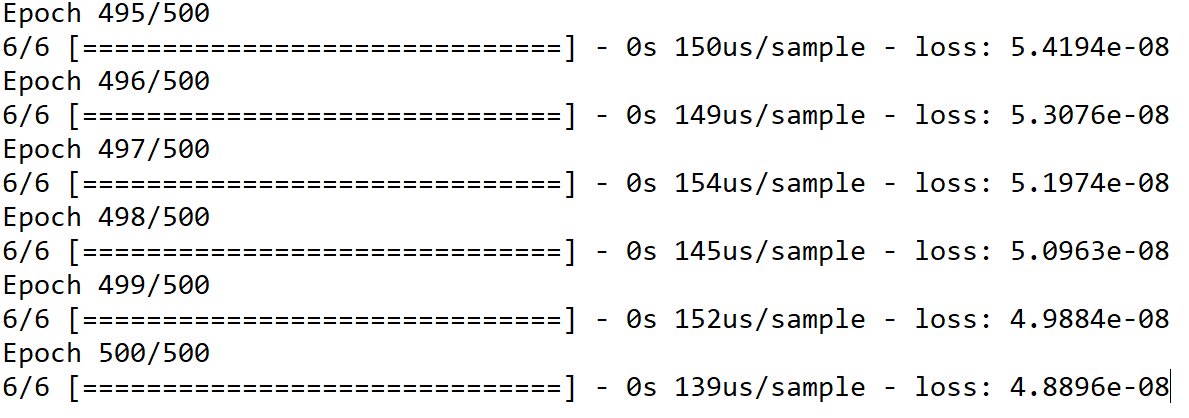You probably don't need all 500 epochs, try experimenting with different values. Looking at this example, the loss is really small after only 50 epochs, so that might be enough!

### Using the model

You now have a model that has been trained to learn the relationship between X and Y.

You can use the `model.predict` method to figure out the Y for an X not previously seen by the model during training. So, for example, if X = 10, what do you think Y will be?

1. Add the following code to the end of `model.py`:

cloud_logger.info(str(model.predict([10.0]))) Note: Your prediction result is passed to `cloud_logger` in order to produce cloud logs which can be checked for progress.
1. Press Ctrl+s or go to`Application Menu` > `File` > `Save`, to save the changes.

2. Take a guess about the Y value, then run your script. Enter the following command in the terminal:

python model.py

The Y value is listed after the training log (epochs).

Example output: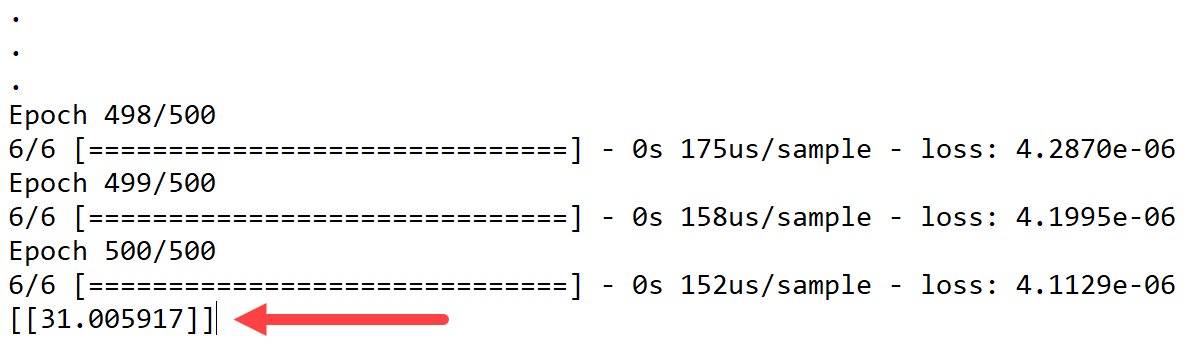You might have thought Y=31, right? But it ended up being a little over (31.005917). Why do you think that is?

Answer: Neural networks deal with probabilities. It calculated that there is a very high probability that the relationship between X and Y is Y=3X+1. But with only 6 data points it can't know for sure. As a result, the result for 10 is very close to 31, but not necessarily 31.

As you work with neural networks, you'll see this pattern recurring. You will almost always deal with probabilities, not certainties, and will do a little bit of coding to figure out what the result is based on the probabilities, particularly when it comes to classification.

Click Check my progress to verify the objective. Use the model

## Congratulations!

This concludes the self-paced lab, TensorFlow: Qwik Start. You created, trained, and tested your own Machine Learning Model using TensorFlow.

This self-paced lab is part of the Intermediate ML: TensorFlow on Google Cloud quest. A quest is a series of related labs that form a learning path. Completing this quest earns you a badge to recognize your achievement. You can make your badge or badges public and link to them in your online resume or social media account. Enroll in this quest and get immediate completion credit. Refer to the Google Cloud Skills Boost catalog for all available quests.

Continue your quest with Predict Housing Prices with TensorFlow and AI Platform, or try one of these suggestions: